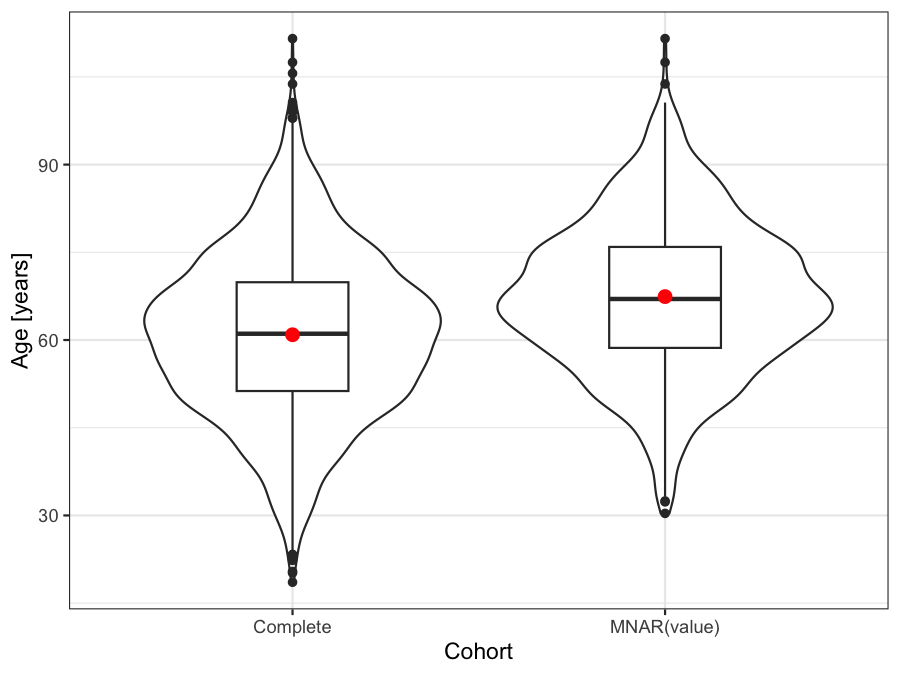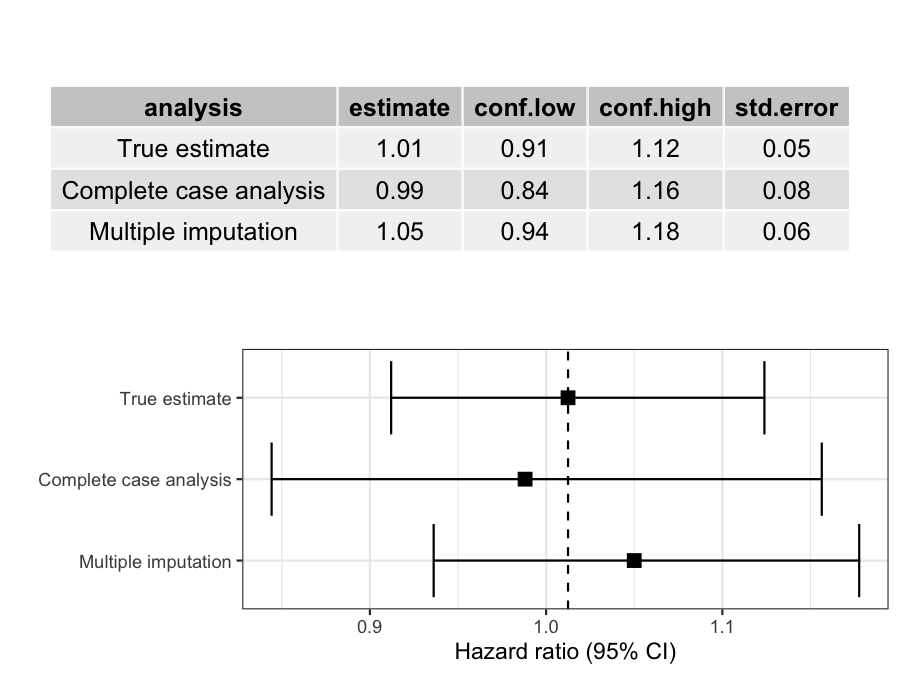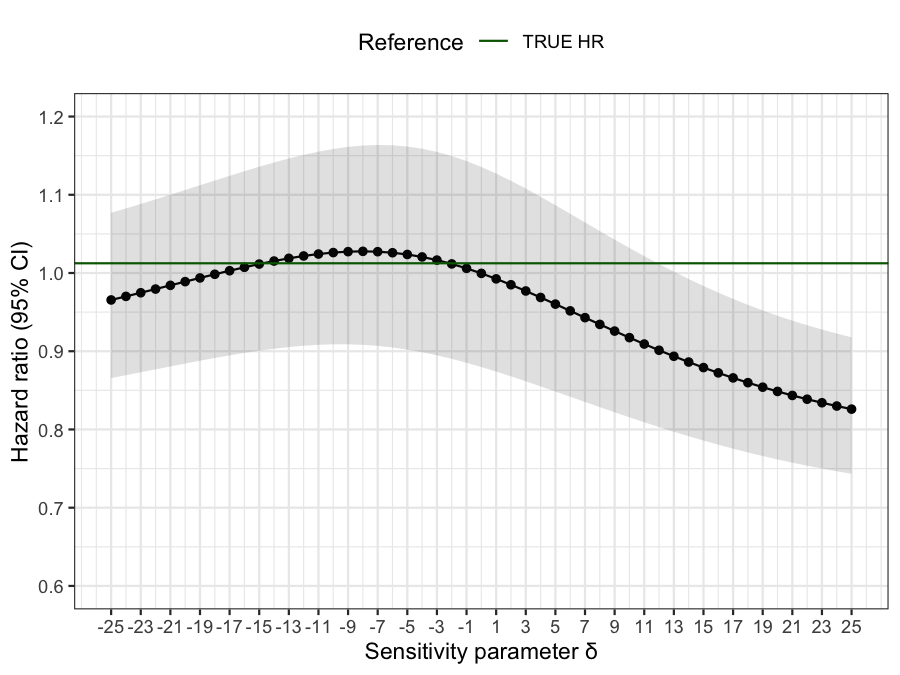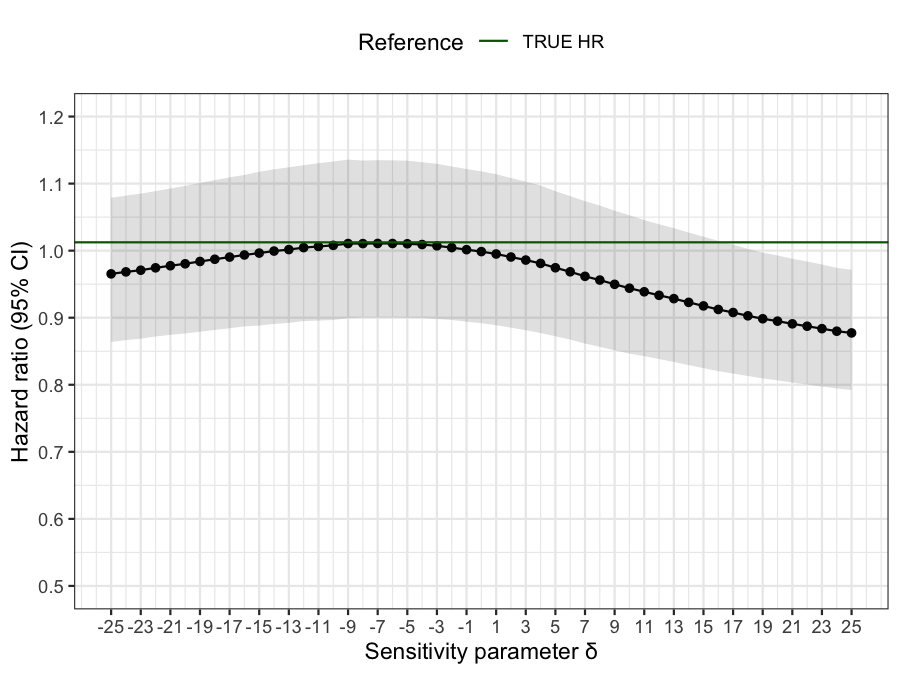# NARFCS sensitivity analysis

# basic setup
library(smdi)
library(ggplot2)
library(survival)
library(gt)
suppressPackageStartupMessages(library(mice))
suppressPackageStartupMessages(library(dplyr))

# Sensitivity analysis for MNAR(value)

As we have seen earlier, if the missingness mechanism of an important covariate, such as a strong partially observed confounder, truly follows either a missing completely at random (MCAR) or a missing not at random value (MNAR[value]) scenario, it may be possible to differentiate these two mechanisms using smdi_diagnose(), but the distinction between the two can also turn out difficult in practice. This can have serious consequences since covariates that follow a true MNAR(value) mechanism may severely bias the effect estimation of an exposure of interest.

In reality, we will not be able to know 100% what the true missingness mechanism is and there may always be some residual uncertainty in case of MNAR(value). On the positive side, however, Leacy and Moreno-Betancur et al. have proposed a useful procedure to impute multivariable missing data under MNAR(value) conditions. By definition, under a MNAR(value) mechanism, the true (but unobserved) values of a variable systematically differ from the observed values. The idea of the not at random fully conditional specification (NARFCS) sensitivity analysis is to specify an imputation model containing a sensitivity parameter 𝛅 that reflects this systematic departure from a missing at random (MAR) mechanism.

## Illustrative example

For example, the age_num of patients below a certain age cut-off may be systematically less frequently recorded. Despite this, age_num may still be an important predictor for the initiation of the treatment of interest and simultaneously an import prognostic variable for the outcome (time to death due to any reason [overall survival] in this example).

For simplicity, we don’t assume any other missing covariates for now. In order to introduce an MNAR(value) mechanism for the age_num covariate, we:

• Determine a missingess pattern, in which only age_num will be set to missing
• Create a weight vector, in which only age_num itself (by a non-zero value) is the linear predictor for the probability of observations becoming missing
• Specify a type of logistic probability distribution for the missingness weights, so that patients with low weighted sum scores (i.e., younger age_num) will have a larger probability of becoming incomplete
• Define an overall missingness proportion of ~40%
# load complete dataset
smdi_data_complete <- smdi_data_complete %>%
fastDummies::dummy_columns(
select_columns = "ses_cat",
remove_most_frequent_dummy = TRUE,
remove_selected_columns = TRUE
)

# determine missingness pattern
age_col <- which(colnames(smdi_data_complete)=="age_num")
miss_pattern <- rep(1, ncol(smdi_data_complete))
miss_pattern_age <- replace(miss_pattern, age_col, 0)

# weights to compute missingness probability
# covariate itself is only predictor
miss_weights_mnar_v <- rep(0, ncol(smdi_data_complete))
miss_weights_mnar_v <- replace(miss_weights_mnar_v, age_col, 1)

miss_prop_age <- .55

set.seed(42)
smdi_data_mnar_v <- ampute(
data = smdi_data_complete,
prop = miss_prop_age,
mech = "MNAR",
patterns = miss_pattern_age,
weights = miss_weights_mnar_v,
bycases = TRUE,
type = "LEFT"
)$amp ## Visual comparison If we plot the direct comparison of age_num distributions between the original data and the data with complete cases after removing those who have a missing value following an MNAR(value) mechanism, we can observe the systematic difference in the two datasets. # plot bind_rows( smdi_data_complete %>% select(age_num) %>% mutate(dataset = "Complete"), smdi_data_mnar_v %>% select(age_num) %>% mutate(dataset = "MNAR(value)") ) %>% ggplot(aes(x = dataset, y = age_num)) + geom_violin() + geom_boxplot(width = 0.3) + stat_summary( fun = "mean", geom = "pointrange", color = "red" ) + labs( y = "Age [years]", x = "Cohort" ) + theme_bw()## smdi diagnostics Now let’s use smdi_diagnose to investigate the three group diagnostics from age_num. This may help characterize the underlying missingness mechanism. smdi_diagnose( data = smdi_data_mnar_v, covar = "age_num", model = "cox", form_lhs = "Surv(eventtime, status)" ) %>% smdi_style_gt() Covariate ASMD (min/max)1 p Hotelling1 AUC2 beta univariate (95% CI)3 beta (95% CI)3 age_num 0.056 (0.004, 0.360) <.001 0.543 -0.41 (95% CI -0.50, -0.32) -0.40 (95% CI -0.49, -0.32) p little: <.001, Abbreviations: ASMD = Median absolute standardized mean difference across all covariates, AUC = Area under the curve, beta = beta coefficient, CI = Confidence interval, max = Maximum, min = Minimum 1 Group 1 diagnostic: Differences in patient characteristics between patients with and without covariate 2 Group 2 diagnostic: Ability to predict missingness 3 Group 3 diagnostic: Assessment if missingness is associated with the outcome (univariate, adjusted) We observe from this diagnostics a median absolute standardized mean difference (ASMD) of 0.056 with a meaningful spread between the minimum ASMD (0.004) and the maximum ASMD (0.360), indicating some differences in patient with and without an observed age value which is in line with the p-value derived by Hotelling’s test. While the ability to predict missingness is rather low, group 3 diagnostic tends to show that even after adjustment, there is a significant difference in the survival of patients with and without an observed age measurement. In total, these characteristics are reminiscent of the diagnostic patterns observed in simulations in case of an MNAR mechanism. Hence, we could suspect that MNAR(value) could be the underlying missingness mechanism, in which case we would want to run some sensitivity analyses to investigate how much an MNAR(value) mechanism could bias our results. ## Comparing treatment effect estimates Since we know the true treatment effect estimate, we can quickly compare and estimate the bias caused by the 40% of MNAR(value) missing values. First, we specify a general outcome model. # outcome model (see data generation script) cox_lhs <- "survival::Surv(eventtime, status)" covariates <- smdi_data_complete %>% select(-c(exposure, eventtime, status)) %>% names() cox_rhs <- paste(covariates, collapse = " + ") cox_form <- as.formula(paste(cox_lhs, "~ exposure +", cox_rhs)) cox_form #> survival::Surv(eventtime, status) ~ exposure + age_num + female_cat + #> ecog_cat + smoking_cat + physical_cat + egfr_cat + alk_cat + #> pdl1_num + histology_cat + copd_cat + ses_cat_1_low + ses_cat_2_middle Next, we compute the true Hazard Ratio (HR), the complete case HR and the standard mice multiple imputation HR. # true outcome model cox_fit_true <- survival::coxph(cox_form, data = smdi_data_complete) %>% broom::tidy(exponentiate = TRUE, conf.int = TRUE) %>% filter(term == "exposure") %>% select(term, estimate, conf.low, conf.high, std.error) %>% mutate(analysis = "True estimate") # complete case analysis cox_fit_cc <- survival::coxph(cox_form, data = smdi_data_mnar_v) %>% broom::tidy(exponentiate = TRUE, conf.int = TRUE) %>% filter(term == "exposure") %>% select(term, estimate, conf.low, conf.high, std.error) %>% mutate(analysis = "Complete case analysis") # Multiple imputation (predictive mean matching) cox_fit_imp <- mice( data = smdi_data_mnar_v, seed = 42, print = FALSE ) %>% with( expr = survival::coxph(formula(paste(format(cox_form), collapse = ""))) ) %>% pool() %>% summary(conf.int = TRUE, exponentiate = TRUE) %>% filter(term == "exposure") %>% select(term, estimate, conf.low = 2.5 %, conf.high = 97.5 %, std.error) %>% mutate(analysis = "Multiple imputation") forest <- bind_rows(cox_fit_true, cox_fit_cc, cox_fit_imp) %>% mutate(analysis = factor(analysis, levels = c("True estimate", "Complete case analysis", "Multiple imputation"))) %>% ggplot(aes(y = forcats::fct_rev(analysis))) + geom_point(aes(x = estimate), shape = 15, size = 3) + geom_errorbar(aes(xmin = conf.low, xmax = conf.high)) + geom_vline(xintercept = cox_fit_true[["estimate"]], linetype = "dashed") + labs(x = "Hazard ratio (95% CI)", y= "") + theme_bw() table <- bind_rows(cox_fit_true, cox_fit_cc, cox_fit_imp) %>% select(-term) %>% relocate(analysis, .before = estimate) %>% mutate(across(tidyselect::where(is.numeric), ~round(.x, 2))) gridExtra::grid.arrange(gridExtra::tableGrob(table, rows = NULL), forest)The deviation of the complete case and multiple imputation estimates aren’t too off which has to do with age_num being a rather moderate confounder in this simulation. Nevertheless, in reality we don’t know this for sure and a sensitivity analysis would probably make sense to test the robustness of our primary analysis. ## NARFCS imputation As mentioned above, under an MNAR(value) mechanism, the true (but unobserved) values of a variable systematically differ from the observed values and this difference is reflected in the sensitivity parameter 𝛅. More specifically, the interpretation of 𝛅 would be the difference in the distribution of missing and observed age_num values conditional on other covariates. Hence, for continuous covariates (like in our example) it would be the difference in mean conditional on female_cat + ecog_cat + smoking_cat + physical_cat + egfr_cat + alk_cat + pdl1_num + histology_cat + copd_cat + ses_cat_1_low + ses_cat_2_middle. If in reality, we would know this sensitivity parameter, we could easily plug it into our NARFCS. ## Tipping point analysis But unfortunately, in reality we usually don’t know the value of 𝛅 and relying on a single 𝛅 may be not too reassuring. Nevertheless, we want to make sure that whatever analytic decision we chose for our primary analysis (be it a complete case approach or an imputation approach), our results would not drastically change if the true underlying missingness mechanism was MNAR(value). This is where the NARFCS sensitivity analysis comes in handy as its strengths as it is also often used as a tipping point analysis, i.e., we model multiple NARFCS-specified imputation models over a range of realistic 𝛅 sensitivity parameters and evaluate if at any pre-specified 𝛅, our confidence interval would cross a certain estimate threshold which would discard the global conclusion of our primary analysis. # initialize method vector method_vector <- rep("", ncol(smdi_data_mnar_v)) # columns for narfcs imputation with sensitivity parameter mnar_imp_method <- which(colnames(smdi_data_mnar_v) == "age_num") # update method vector method_vector <- replace(x = method_vector, list = c(mnar_imp_method), values = c("mnar.norm")) # modeled over a range of deltas narfcs_modeled <- function(i){ # mnar model specification ('i' is delta parameter) mnar_blot <- list(age_num = list(ums = paste(i))) narfcs_imp <- mice( data = smdi_data_mnar_v, method = method_vector, blots = mnar_blot, seed = 42, print = FALSE ) %>% with( expr = survival::coxph(formula(paste(format(cox_form), collapse = ""))) ) %>% pool() %>% summary(conf.int = TRUE, exponentiate = TRUE) %>% filter(term == "exposure") %>% select(term, estimate, conf.low = 2.5 %, conf.high = 97.5 %, std.error) %>% mutate(delta = i) } narfcs_range <- lapply( X = seq(-25, 25, 1), FUN = narfcs_modeled ) narfcs_range_df <- do.call(rbind, narfcs_range) reference_lines <- tibble::tibble( yintercept = c(cox_fit_true[]), Reference = c("TRUE HR"), color = c("darkgreen") ) narfcs_range_df %>% ggplot(aes(x = delta, y = estimate)) + geom_point() + geom_line() + geom_ribbon(aes(ymin = conf.low, ymax = conf.high), alpha = 0.15) + labs(x = "Sensitivity parameter δ", y = "Hazard ratio (95% CI)") + scale_x_continuous(breaks = seq(-25, 25, 2), limits = c(-25, 25)) + scale_y_continuous(breaks = seq(0.6, 1.2, 0.1), limits = c(0.6, 1.2)) + geom_hline(aes(yintercept = yintercept, color = Reference), reference_lines) + scale_colour_manual(values = reference_lines$color) +
theme_bw() +
theme(legend.position="top")Based on this tipping point analysis, the systematic difference between observed and unobserved age_num values would have needed to be so drastic that a significant departure of our primary analysis is rather implausible. This is also supported by the fact that age_num is a rather weak to moderate confounder and complete case and non-NARFCS multiple imputation results may have been different, had age_num been a stronger prognostic factor.

# Multivariate missingness PD-L1 biomarker example

Now, we can do the same in our missing smdi_data dataset. However, this gets a little tricky since we have multiple missing covariates, each of which follow different missingness mechanisms. Here is again a quick overview of the missing variables using the smdi_summarize() function.

smdi::smdi_data %>%
smdi::smdi_summarize()
#> # A tibble: 3 × 4
#>   covariate n_miss prop_miss prop_miss_label
#>   <chr>      <int>     <dbl> <chr>
#> 1 egfr_cat    1015      40.6 40.60%
#> 2 ecog_cat     899      36.0 35.96%
#> 3 pdl1_num     517      20.7 20.68%

## NARFCS imputation

The NARFCS procedure that comes with the mice package provides the flexibility to execute the NARFCS procedure in the presence of other partially observed covariates which may be assumed missing at random.

Given the smdi_diagnose analysis, we hypothesize that pdl1_num may be MNAR(value). This is plausible in the context that the pdl1_num expression of patients who had been tested before and showed lower PD-L1 staining levels are less likely to be tested in the future. Despite this, pdl1_num is still an important predictor for the initiation of the treatment of interest and simultaneously an import prognostic variable for the outcome (time to death due to any reason [overall survival]. Hence, we decide to run a tipping point sensitivity analysis by imputing pdl1_num over a range of 𝛅 sensitivity parameters while imputing ecog_cat and egfr_cat under a “normal” imputation model.

# we one hot encode the ses_cat variable again
# in the smdi_data dataset
smdi_data <- smdi::smdi_data %>%
fastDummies::dummy_columns(
select_columns = "ses_cat",
remove_most_frequent_dummy = TRUE,
remove_selected_columns = TRUE
)

# initialize method vector
method_vector <- rep("", ncol(smdi_data))

# specify columns for narfcs and 'normal' imputation
pdl1_mnar_col <- which(colnames(smdi_data) == "pdl1_num")
ecog_mar_col <- which(colnames(smdi_data) == "ecog_cat")
egfr_mnar_col <- which(colnames(smdi_data) == "egfr_cat")

# update method vector
method_vector <- replace(
x = method_vector,
list = c(pdl1_mnar_col, ecog_mar_col, egfr_mnar_col),
values = c("mnar.norm", "logreg", "logreg")
)

# modeled over a range of deltas
narfcs_modeled <- function(i){

# mnar model specification for pdl1_num ('i' is delta parameter)
mnar_blot <- list(pdl1_num = list(ums = paste(i)))

narfcs_imp <- mice(
data = smdi_data,
method = method_vector,
blots = mnar_blot,
seed = 42,
print = FALSE
) %>%
with(
expr = survival::coxph(formula(paste(format(cox_form), collapse = "")))
) %>%
pool() %>%
summary(conf.int = TRUE, exponentiate = TRUE) %>%
filter(term == "exposure") %>%
select(term, estimate, conf.low = 2.5 %, conf.high = 97.5 %, std.error) %>%
mutate(delta = i)

}

narfcs_range <- lapply(
X = seq(-25, 25, 1),
FUN = narfcs_modeled
)

narfcs_range_df <- do.call(rbind, narfcs_range)

reference_lines <- tibble::tibble(
yintercept = c(cox_fit_true[]),
Reference = c("TRUE HR"),
color = c("darkgreen")
)

narfcs_range_df %>%
ggplot(aes(x = delta, y = estimate)) +
geom_point() +
geom_line() +
geom_ribbon(aes(ymin = conf.low, ymax = conf.high), alpha = 0.15) +
labs(x = "Sensitivity parameter δ", y = "Hazard ratio (95% CI)") +
scale_x_continuous(breaks = seq(-25, 25, 2), limits = c(-25, 25)) +
scale_y_continuous(breaks = seq(0.5, 1.2, 0.1), limits = c(0.5, 1.2)) +
geom_hline(aes(yintercept = yintercept, color = Reference), reference_lines) +
scale_colour_manual(values = reference_lines\$color) +
theme_bw() +
theme(legend.position="top")As in the example above, pdl1_num is sensitive to departures from MAR, but results would only change under unrealistically strong differences. In fact, the point estimates perfectly align with the true estimate by sliding 𝛅 a bit to the left of the range scale. The interpretation would be that patients with lower pdl1_num values are systematically less likely to be observed conditional on all other covariates - which is exactly what we simulated.